LTE Quick Reference

CDD (Cyclic Delay Diversity)

CDD is a kind of transmit diversity mechanism implemented by applying a different phase delay (cyclic phase delay) for each OFDM subcarrier. It is used in spatial multiplexing to increase diversity between the 2 spatial paths.

Very simply put, in CDD... one antenna is transmitting the original copy of data and the other antenna is transmitting the cyclic shifted version of the original data as illustrated below (see how the yellow part to represent the cyclic shift)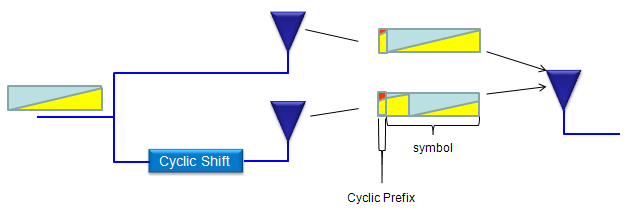If you represent the transmitted data in frequecy domain, the original data and the cyclic shifted version can be represented as follows. As you see, the cyclic shif in time domain produce the phase shift for each symbols in frequency domain and it generate the same effect as frequency diversity.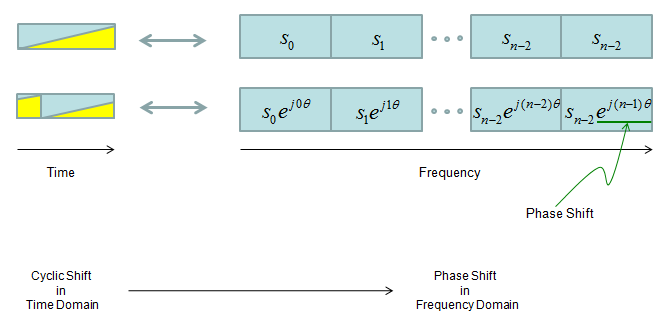CDD is applied differently for each transmission mode as shown in the following table.

 TM type Number of Antenna CDD Type TM1 1 NO CDD TM2 2 NO CDD TM3 2 LARGE CDD TM4 2 NO CDD TM5 2 NO CDD TM6 2 NO CDD TM7 1 NO CDD

What is the difference between NO CDD (without CDD) and LARGE CDD ? To be honest, I don't think I can explain it in easy way implying that even I don't have fully-detailed understanding on this. If I don't explain anything in plain term. You can refer to 36.211 section 6.3.4.2 Precoding for spatial multiplexing or you can refer to the precoding section of LTE Basic Procedure page. (Sometimes there is some concept that you cannot understand without going through the mathmatical description and pulling your hair for long time).

If you look at the following illustration, CDD is implemented by applying two additional matrix (D, U) to channel matrix (W) in precoding process. CDD being appiled in Precoding Step implies that real implementation of CDD is done in frequency domain and will be converted to Time Domain right before being transmitted through Antenna.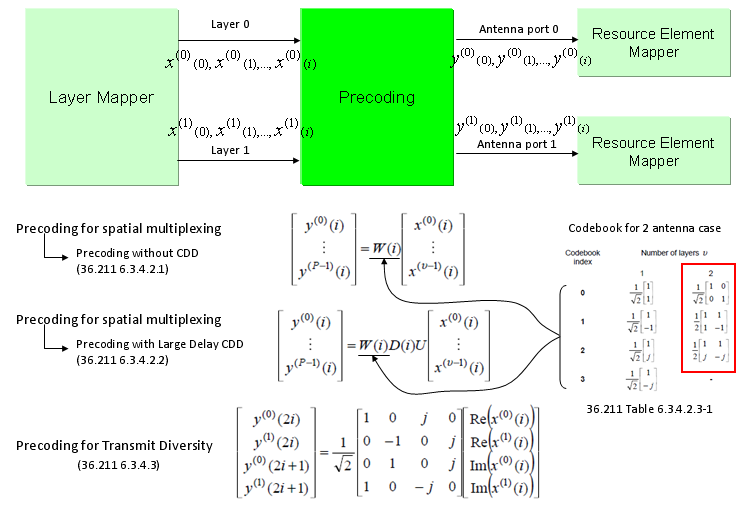If you look into the CDD part of precoding, the matrix are as follows. If you see the D(i) matrix, it has all zero value except diagonal line. The values on diagonal line performs phase shift.I know not so many people want to do math, but sometimes it would be easier to clarify many things if you do some math.

If you plug 2x2 CDD matrix into Precoding equation, you would get a format as shown below. (I just obmitted W(i) part for simplicity. It is just doing scaling without any phase shift or rotation). Just try to understand overall function of Matrix D(i) and U as commented below and be aware that D(2,2) of D(i) matrix gets toggled between +1 and -1 depending on whether 'i' is odd or even.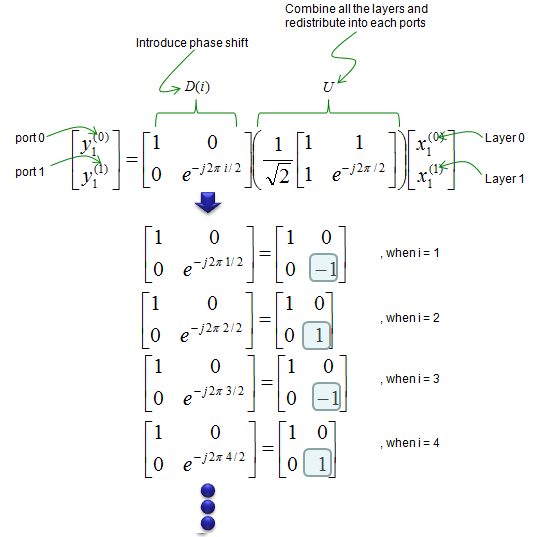If you want to clarify even further, expand the matrix equation and analyze the resulting equation as shown below.

If you multiply symbols from Layer Mapper with CDD matrix, you would easily notice how each of the symbols get combined and allocated to each of the antenna. Notice that the result gets different depending on whether the index of symbols are odd or even.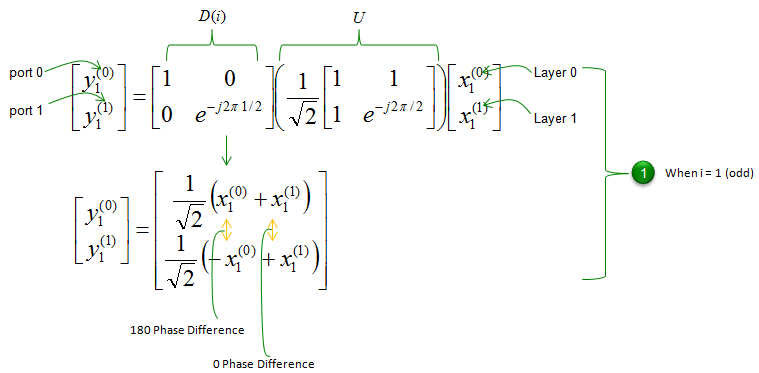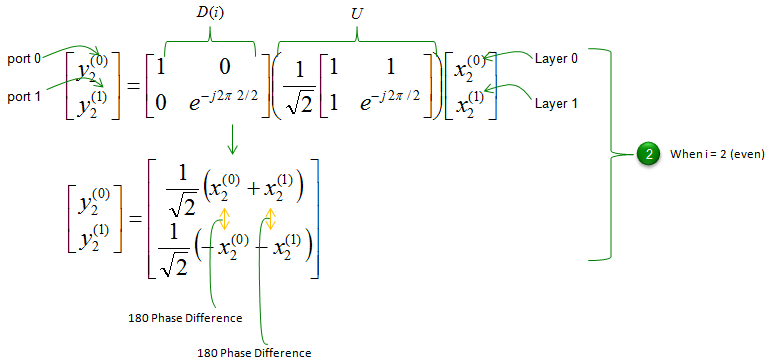Following is some facts you can directly read out from the equations listed above.

i) Each antenna port (port 0 and port 1) transmit the portions from the both layers. (Symbols from the two layers are linearly combined and redistributed between two antenna port)

i) The phase difference of layer 1 data between Antenna 0 and Antenna 1 is 180 or 0 depending on i.

ii)  The phase difference of layer 0 data between Antenna 0 and Antenna 1 is 0 or 180 depending on i.

iii) The phase difference of layer 0 and 1 data between odd numbered symbol and even numbered symbol is 180 on Antenna port 1.

iv) The phase difference of layer 0 and 1 data between odd numbered symbol and even numbered symbol is 0 on Antenna port 0.

The above facts of i) and ii) tell us that the same signal ( layer 0 or 1 data ) is transmitted from the two Tx-antenna ports with the phase relation changed every subcarrier by 180-degree step.